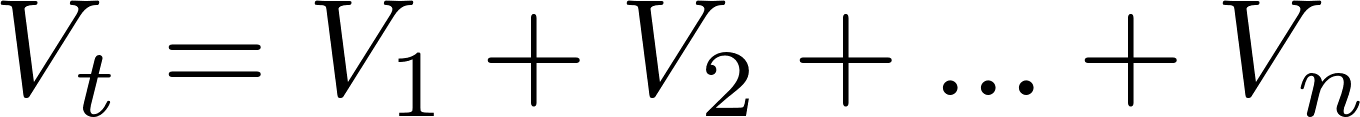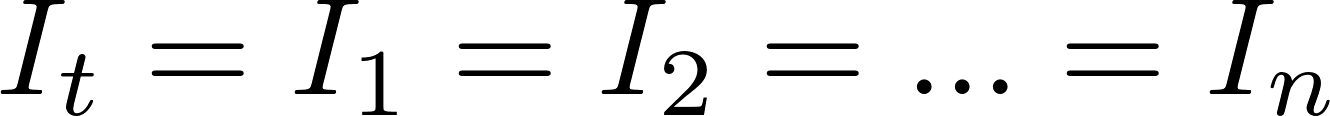# Resistors in series

Resistors can be connected in series to increase the total resistance. The resistance and the voltage are equal to the sum of the values of all individual resistors and the current is the same for all resistors.

### Formulas

The total voltage, current, and resistance are calculated like this: